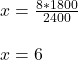## Daily income of 8 workers is Rs. 2400. How many workers will earn Rs.1800

Question

Daily income of 8 workers is Rs. 2400. How many workers will earn Rs.1800

in progress 0
4 weeks 2021-08-18T11:17:06+00:00 2 Answers 1 views 0

6 workers will earn Rs. 1800.

Step-by-step explanation:

Given :-

• Daily income of 8 workers is Rs. 2400.

To find :

• How many workers will earn Rs.1800.

Solution :-

Let

no. of workers will earn Rs. 1800 = x.

We need to write a ratio to solve.

• 8 = 2400
• x = 1800

using cross products

• 8 × 1800 = x × 2400
• 14400 = 2400x

Divide each side by 2400

• 14400/2400 = 2400x/2400
• 6 = x

Hence, 6 workers will earn Rs. 1800.

6 workers

Step-by-step explanation:

Number workers to earnings is direct proportion.

8: 2400 :: x : 1800

Product of means = Product of extremes

2400 * x = 8*1800